# Surface Area Of Prisms Worksheet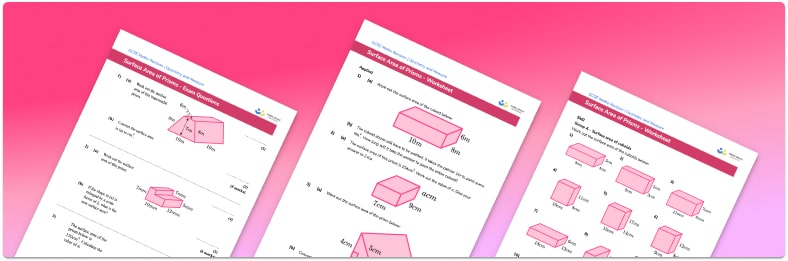• Section 1 of the surface area of prisms worksheet contains 27 skills-based surface area of prisms questions, in 3 groups to support differentiation
• Section 2 contains 4 applied surface area of prisms questions with a mix of word problems and deeper problem solving questions
• Section 3 contains 3 foundation and higher level GCSE exam style surface area of prisms questions
• Answers and a mark scheme for all surface area of prisms questions are provided
• Questions follow variation theory with plenty of opportunities for learners to work independently at their own level
• All questions created by fully qualified expert secondary maths teachers

Suitable for GCSE maths revision for AQA, OCR and Edexcel exam boards

• This field is for validation purposes and should be left unchanged.

You can unsubscribe at any time (each email we send will contain an easy way to unsubscribe). To find out more about how we use your data, see our privacy policy.

### Surface area of prisms at a glance

Surface area is a measure of the total area of all of the faces of a solid shape. A prism is a 3D shape with identical polygons at each end, connected by a number of rectangular faces. To find the total surface area of a prism, we find the area of each face and add them together.

For example, a triangular prism has two triangular ends connected by three rectangular sides. To find the total surface area of the triangular prism, we find the area of the two triangles. We then find the area of the three sides (sometimes called the lateral surface area) and add these to the area of the triangles to give the total surface area.

Surface area of 3D shapes is measured in square units such as cm^2 or mm^2 .

Looking forward, students can then progress to additional surface area worksheets and other geometry worksheets, for example a simplifying expressions worksheet or simultaneous equations worksheet.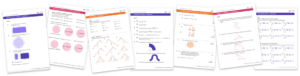For more teaching and learning support on Geometry our GCSE maths lessons provide step by step support for all GCSE maths concepts.

## Related worksheets

Surface Area Of A Triangular Prism Worksheet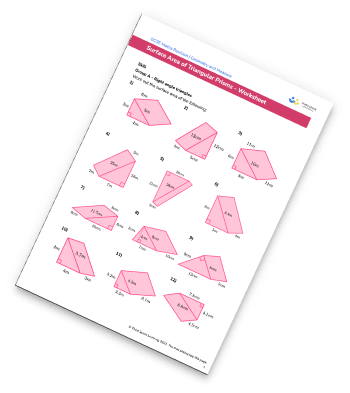Surface Area Of Cones Worksheet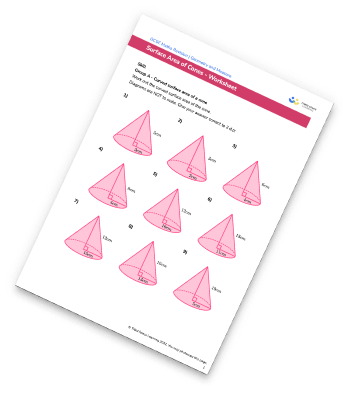Surface Area Of Cylinders Worksheet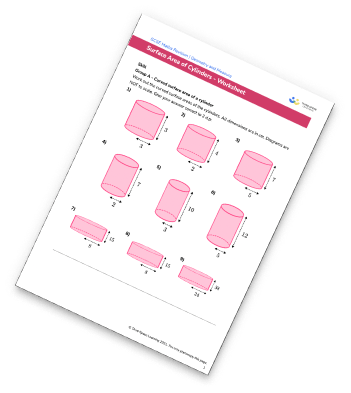Surface Area Of A Cuboid Worksheet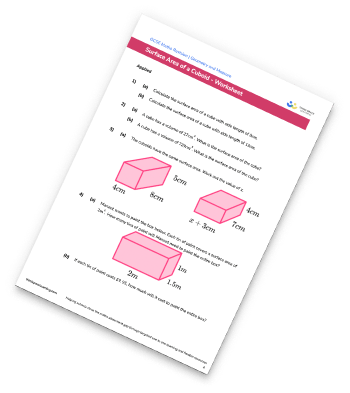## Do you have KS4 students who need more focused attention to succeed at GCSE?There will be students in your class who require individual attention to help them succeed in their maths GCSEs. In a class of 30, it’s not always easy to provide.

Help your students feel confident with exam-style questions and the strategies they’ll need to answer them correctly with our dedicated GCSE maths revision programme.

Lessons are selected to provide support where each student needs it most, and specially-trained GCSE maths tutors adapt the pitch and pace of each lesson. This ensures a personalised revision programme that raises grades and boosts confidence.

Find out more